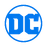## FANDOM

109,595 PagesThis page contains a listing of all known appearances of Amon Hakk (New Earth).
If you find an Issue this Character appears in that is not shown here, please edit that issue adding "Amon Hakk (New Earth)" as a Cast member.

All items (65)

D
•Darkstars Vol 1 11
•Darkstars Vol 1 12
•DCU: Trinity Vol 1 2
G
•Green Lantern Vol 3 45
•Guy Gardner: Warrior Vol 1 29
L
•L.E.G.I.O.N. Vol 1 30
•L.E.G.I.O.N. Vol 1 31
•L.E.G.I.O.N. Vol 1 32
•L.E.G.I.O.N. Vol 1 34
•L.E.G.I.O.N. Vol 1 35
•L.E.G.I.O.N. Vol 1 36
•L.E.G.I.O.N. Vol 1 37
•L.E.G.I.O.N. Vol 1 38
•L.E.G.I.O.N. Vol 1 39
•L.E.G.I.O.N. Vol 1 40
•L.E.G.I.O.N. Vol 1 42
•L.E.G.I.O.N. Vol 1 43
•L.E.G.I.O.N. Vol 1 44
•L.E.G.I.O.N. Vol 1 48
•L.E.G.I.O.N. Vol 1 49
•L.E.G.I.O.N. Vol 1 50
•L.E.G.I.O.N. Vol 1 52
•L.E.G.I.O.N. Vol 1 53
•L.E.G.I.O.N. Vol 1 54
•L.E.G.I.O.N. Vol 1 55
•L.E.G.I.O.N. Vol 1 57
•L.E.G.I.O.N. Vol 1 58
•L.E.G.I.O.N. Vol 1 59
•L.E.G.I.O.N. Vol 1 62
•L.E.G.I.O.N. Vol 1 66
•L.E.G.I.O.N. Vol 1 68
•L.E.G.I.O.N. Vol 1 69
•L.E.G.I.O.N. Vol 1 70
R
•R.E.B.E.L.S. Vol 1 0
•R.E.B.E.L.S. Vol 1 8
•R.E.B.E.L.S. Vol 1 13
•R.E.B.E.L.S. Vol 1 14
•R.E.B.E.L.S. Vol 1 16
•R.E.B.E.L.S. Vol 1 17
•R.E.B.E.L.S. Vol 2 1
•R.E.B.E.L.S. Vol 2 2
•R.E.B.E.L.S. Vol 2 4
•R.E.B.E.L.S. Vol 2 5
•R.E.B.E.L.S. Vol 2 6
•R.E.B.E.L.S. Vol 2 7
•R.E.B.E.L.S. Vol 2 8
•R.E.B.E.L.S. Vol 2 9
•R.E.B.E.L.S. Vol 2 10
•R.E.B.E.L.S. Vol 2 11
•R.E.B.E.L.S. Vol 2 12
•R.E.B.E.L.S. Vol 2 13
•R.E.B.E.L.S. Vol 2 14
•R.E.B.E.L.S. Vol 2 15
•R.E.B.E.L.S. Vol 2 16
•R.E.B.E.L.S. Vol 2 17
•R.E.B.E.L.S. Vol 2 18
•R.E.B.E.L.S. Vol 2 19
•R.E.B.E.L.S. Vol 2 21
•R.E.B.E.L.S. Vol 2 22
•R.E.B.E.L.S. Vol 2 23
•R.E.B.E.L.S. Vol 2 25
•R.E.B.E.L.S. Vol 2 26
•R.E.B.E.L.S. Vol 2 27
•R.E.B.E.L.S. Vol 2 28
•R.E.B.E.L.S. Annual Vol 2 1
Community content is available under CC-BY-SA unless otherwise noted.